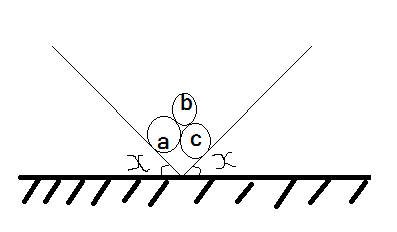# Precarious cylindersThree identical rigid circular cylinders A , B and C are arranged on smooth inclined surfaces as shown.

The least value of $x$ that prevent the arrangement from collapsing can be expressed as $\tan^{-1} \dfrac a{3^{c/d}}$, where $a,c$ and $d$ are positive integers with $c,d$ coprime.

Submit your answer as $a+c+d$.

×